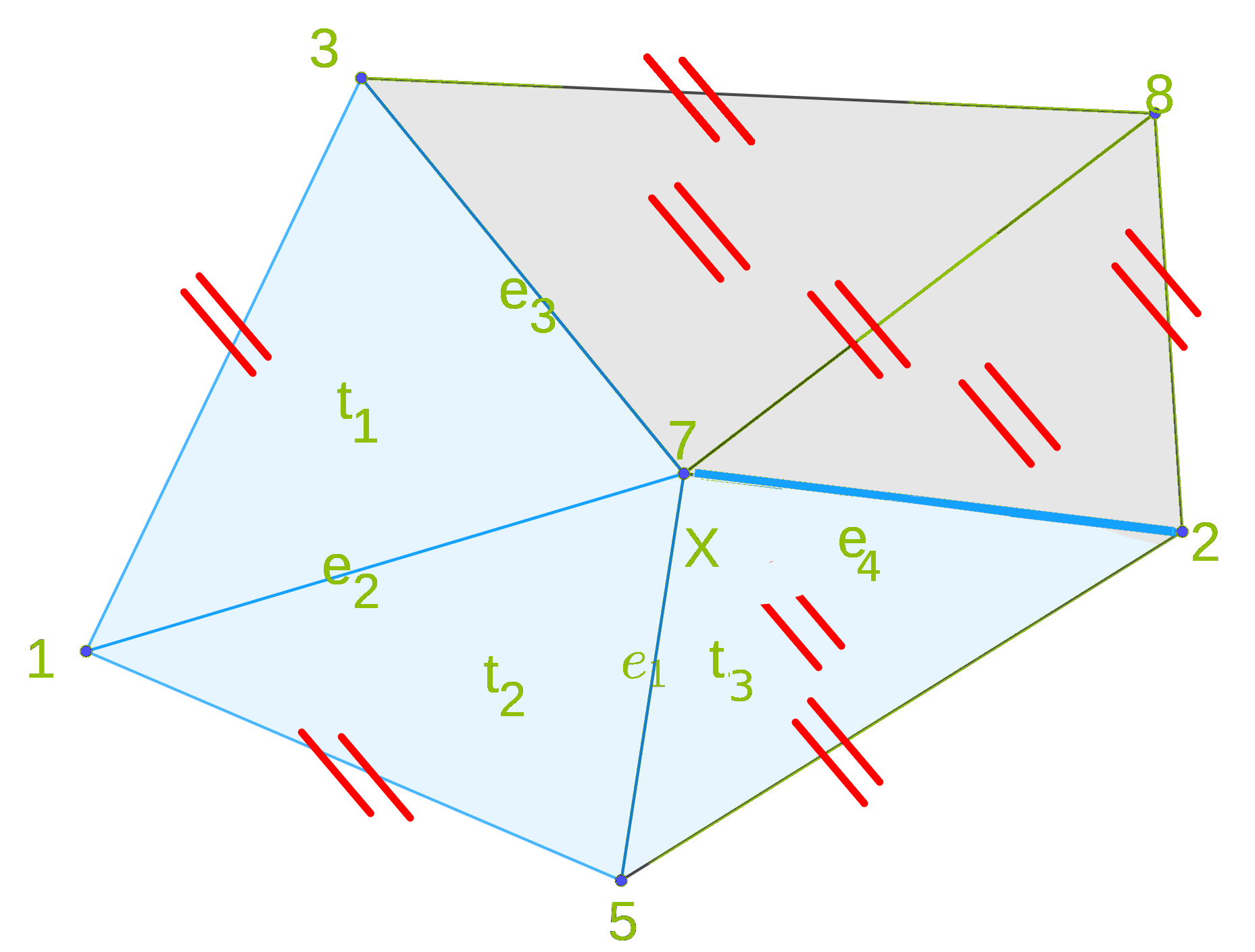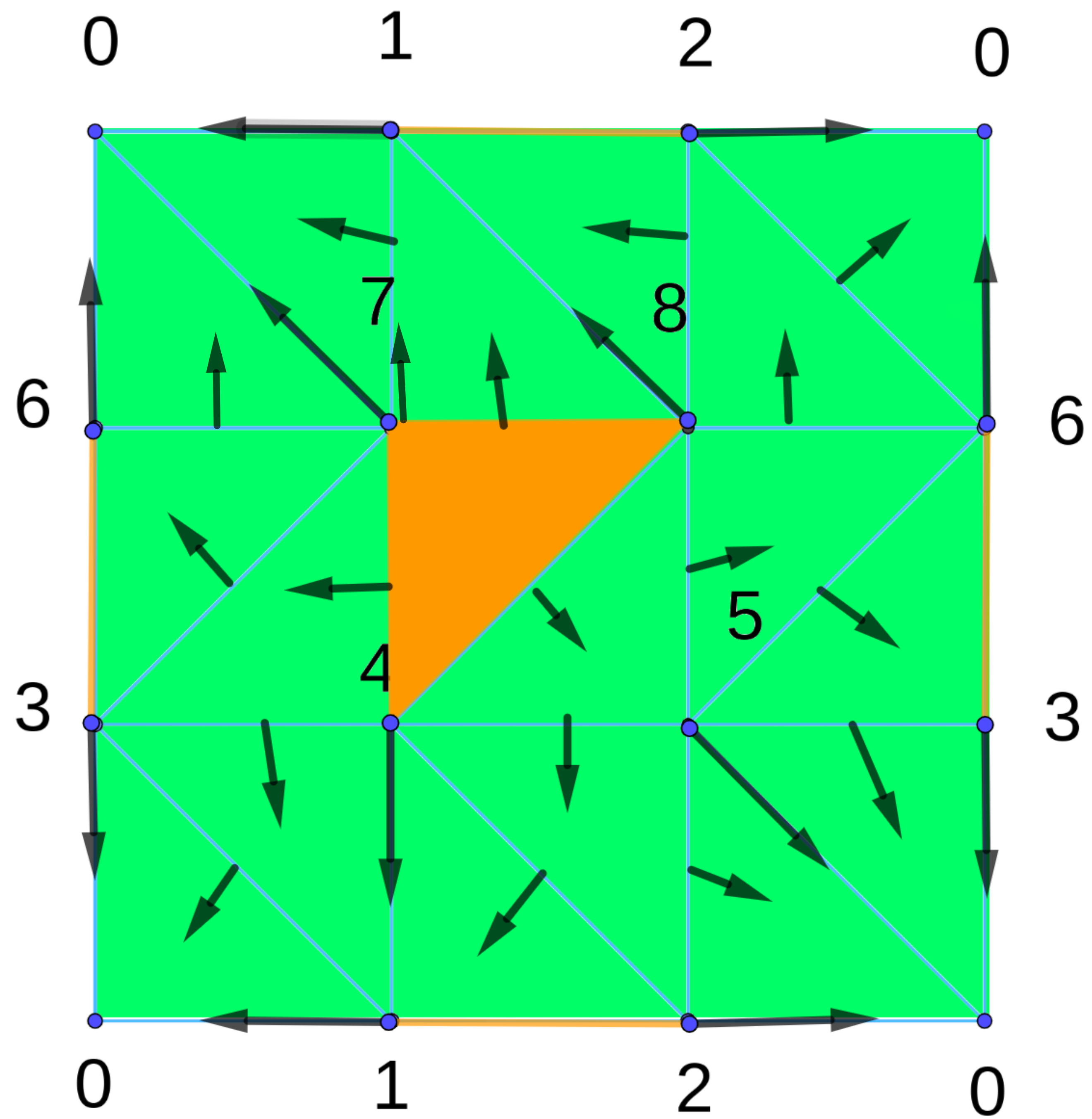Here we look at an algorithm that creates a discrete Morse function on a simplicial complex with values defined on its vertices. The algorithm finds the critical points that match the changes in topology between successive elements of a filtration.

# Requirments of algorithm

The algorithm processes the lower star of vertices of a simplicial complex. A lower star of a vertex x in a simplicial complex $\mathcal{K}$ with scalar value g defined on vertices of $\mathcal{K}$ is the set of all simplices containing x as a vertex and if $\beta$ is a simplex containing x as a vertex then the scalar value of x is greater than or equal to the scalar value of any other vertex of $\beta$. Here is the mathematical definition of lower star set:
$L(x)=\{\beta \in \mathcal{K} \mid x \in \beta \: and \: \max\limits_{v \in \beta} \: g(v) \leq g(x)\}$Lower Star set of the vertex x=7. L(x)={e1,e2,e3,e4,t1,t2,t3,} The algorithm requires an ordering, $G(\alpha)$ of each lower star. The used ordering is the Lexicographic order . It is the way of ordering of words based on the alphabetical order of their component letters. For example, $234 < 45 < 456 < 9$ is lexicographic ordering. The following is the lower star of each vertex of triangulation of the torus in the previous chapter in lexicographic order.
$L(0)=()$
$L(1)=(\overline{10})$
$L(2)=(\overline{20},\overline{21})$
$L(3)=(\overline{30},\overline{31},\bigtriangleup(310))$
$L(4)=(\overline{41},\overline{42},\bigtriangleup(421), \overline{43},\bigtriangleup(431))$
$L(5)=(\overline{50},\overline{52},\bigtriangleup(520),\overline{53},\bigtriangleup(530),\overline{54},\bigtriangleup(542))$
$L(6)=(\overline{60},\overline{62},\bigtriangleup(620),\overline{63},\overline{65},\bigtriangleup(653))$
$L(7)=(\overline{70},\overline{71},\bigtriangleup(710),\overline{73},\overline{74},\bigtriangleup(743),\overline{76},\bigtriangleup(760),\bigtriangleup(763))$
$L(8)=(\overline{81},\overline{82},\bigtriangleup(821),\overline{84},\overline{85},\bigtriangleup(854),\overline{86},\bigtriangleup(862),$
$\bigtriangleup(865),\overline{87},\bigtriangleup(871))$
Notice, that the vertices are labeled by their scalar value $g$. Other cells are labeled by their vertices in decreasing order, for example a cell $\alpha =\{1,2,3\}$ will be labeled (321) and can not be labeled in other way.

# The algorithmThe algorithm iterates over the vertices of the simplicial complex. The order of processing the vertices is not important. The algorithm finds the lower star of the currently processed vertex. If the set of lower star is empty, then this vertex is local minimum. If the set contains other simplices, then the vertex is paired with the lowest incident edge (here we use lexicographic ordering). Then we find the lowest cell $\alpha^p$ which is not paired and try to pair it with the lowest higher cell $\beta^{p+1}$ which also is not paired. We repeat this process to pair every cell in lower star with higher-dimensional cell. If a cell can not be paired, then this cell is critical. To achieve the pairing and ordering of the cells, the algorithm uses two priority queues: PQzero which always contains all cells that have no more free faces and PQone which contains all cells that have exactly one free face left. The cells in these priority queues are ordered by the lexicographic order. The algorithm also requires the function num_unpaired_faces($\alpha$) which returns the number of faces of $\alpha$ that are in the lower star set and are not paired with other cells nor critical. When there is a single available unpaired face for the cell $\alpha$, we call this face pair($\alpha$).

# Example

We process the lower star of each vertex (the order does not matter). We begin with vertex 0. $L(0) =\{\}$ so 0 will be added to critical points. Now, we process vertex 1. $L(1)=(\overline{10})$, we pair 1 with $\overline{10}$ and so L(1) is done. Then we process vertex 2. $L(2)=(\overline{20},\overline{21})$ vertex 2 is paired with edge $\overline{20}$ and edge $\overline{21}$ is added to PQzero. PQone is empty. Since PQzero contains only $\overline{21}$, so it will be added to critical cells. So L(2) is done. $L(3)=(\overline{30},\overline{31},\bigtriangleup(310))$. The vertex 3 will be paired with edge $\overline{30}$. The edge $\overline{31}$ will be add to PQzero since its face in lower star has been paired (we consider only faces according lower star, here face 3 face 0 does not apply) and $\bigtriangleup(310)$ will be inserted in PQone since it has only one unpaired face, namely $\overline{31}$. Now, we iterate in PQone and remove $\bigtriangleup(310)$ and pair it with its pair $\overline{31}$. Repeating the algorithm on all other vertices, we will get the the critical cell list $C = \{0, \overline{21}$,$\overline{63}$,$\bigtriangleup(874)\}$that match our expectations and the discrete vector field $V = \{1:\overline{10},2:\overline{20}, 3: \overline{30},4:\overline{41},5:\overline{40},6:\overline{60},7:\overline{70},8:\overline{81} ,\overline{31}:\bigtriangleup(310),\overline{43}:\bigtriangleup(431),\overline{42}:\bigtriangleup(421),\overline{54}:\bigtriangleup(542),\overline{52}:\bigtriangleup(520),\overline{53}:\bigtriangleup(530),\overline{73}:\bigtriangleup(763),\overline{74}:\bigtriangleup(743),\overline{84}:\bigtriangleup(854),\overline{85}:\bigtriangleup(865),\overline{65}:\bigtriangleup(653),\overline{76}:\bigtriangleup(760),\overline{71}:\bigtriangleup(710),\overline{87}:\bigtriangleup(871),\overline{82}:\bigtriangleup(821),\overline{86}:\bigtriangleup(862),\overline{62}:\bigtriangleup(620)\}$. The illustration of critical cells and discrete vector field are shown in next figure.